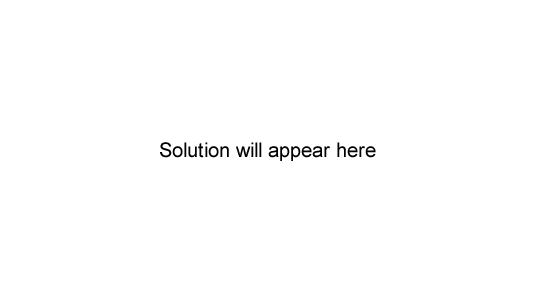Redox reactions 2007 VCE Sulfur dioxide (SO2) is a chemical of major industrial significance. a. SO2 gas can be produced in a reaction between concentrated sulfuric acid and nickel metal. A solution containing Ni2+ ions is also formed. Write balanced equations for the i. oxidation reaction Solution ii. reduction reaction Solution iii. overall reaction, showing the states of all reactants and products. SolutionSO2 can also be produced in a chemical reaction between zinc sulÞ te (ZnSO3) and hydrochloric acid according to the equation ZnSO3(s) + 2H+(aq) → Zn2+(aq) + SO2(g) + H2O(l) Is this reaction also a redox reaction? Explain your answer. Solution Which one of the following is least likely to be a product of a redox reaction between sulfuric acid and zinc metal? A. H2 B. H2S C. SO2 D. SO3 Solution